## TABE Math Formulas

If you are looking for a complete list of all math formulas, they are available in the TABE® Mathematics test's formula sheet. The first and foremost thing is to understand that this test contains a sheet which displays formulas relating to geometric measurement. The TABE test will provide you with formulas to ensure that you can focus on application rather than memorization. However, the test does not offer a complete list of all basic formulas for this exam. This means that in order to be prepared for the examination, it is best if you are able to recall some math formulas from memory.

Math formulas are constantly reviewed and applied throughout the year. It's a good idea to stock up on the most commonly used ones for those days when you might forget them! These will be beneficial during the test day, as well as other math topics that are covered in school. Remember how to use these formulas when using them or giving examples!

### The Most Comprehensive TABE Math Preparation Bundle

$76.99$36.99
52% Off*

Includes TABE Math Prep Books, Workbooks, and Practice Tests

##### Place Value

The value of the place, or position, of a digit in a number.
Example: In 456, the 5 is in “tens” position.

##### Fractions

A number expressed in the form $$\frac{a}{b}$$
Adding and Subtracting with the same denominator:
$$\frac{a}{b}+\frac{c}{b}=\frac{a+c}{b}$$
$$\frac{a}{b}-\frac{c}{b}=\frac{a-c}{b}$$
Adding and Subtracting with the different denominator:
$$\frac{a}{b}+\frac{c}{d}=\frac{ad+cb}{bd}$$
$$\frac{a}{b}-\frac{c}{d}=\frac{ad-cb}{bd}$$
Multiplying and Dividing Fractions:
$$\frac{a}{b} × \frac{c}{d}=\frac{a×c}{b×d}$$
$$\frac{a}{b} ÷ \frac{c}{d}=\frac{\frac{a}{b}}{\frac{c}{d}}=\frac{ad}{bc}$$

##### Comparing Numbers Signs

Equal to $$=$$
Less than $$<$$
Greater than $$>$$
Greater than or equal $$≥$$
Less than or equal $$≤$$

##### Rounding

Putting a number up or down to the nearest whole number or the nearest hundred, etc.
Example: 64 rounded to the nearest ten is 60 , because 64 is closer to 60 than to 70.

##### Whole Number

The numbers $$\{0,1,2,3,…\}$$

##### Estimates

Find a number close to the exact answer.

##### Decimals

Is a fraction written in a special form. For example, instead of writing  $$\frac{1}{2}$$ you can write $$0.5$$.

##### Mixed Numbers

A number composed of a whole number and fraction. Example: $$2 \frac{2}{ 3}$$ Converting between improper fractions and mixed numbers: $$a \frac{c}{b}=a+\frac{c}{b}= \frac{ab+ c}{b}$$

##### Factoring Numbers

Factor a number means to break it up into numbers that can be multiplied together to get the original number. Example:$$12=2×2×3$$

##### Divisibility Rules

Divisibility means that you are able to divide a number evenly. Example: 24 is divisible by 6, because $$24÷6=4$$

##### Greatest Common Factor

Multiply common prime factors
Example:$$200=2×2×2×5×5 60=2×2×3×5$$
GCF $$(200,60)=2×2×5=20$$

##### Least Common Multiple

Check multiples of the largest number
Example: LCM (200, 60): 200 (no),  400 (no), 600 (yes!)

##### Integers

$$\{…,-3,-2,-1,0,1,2,3,…\}$$
Includes: zero, counting numbers, and the negative of the counting numbers

##### Real Numbers

All numbers that are on number line. Integers plus fractions, decimals, and irrationals  etc.) ($$\sqrt{2},\sqrt{3},π$$, etc.)

##### Order of Operations

PEMDAS
(parentheses / exponents / multiply / divide / add / subtract)

##### Absolute Value

Refers to the distance of a number from , the distances are positive as absolute value of a number cannot be negative. $$|-22|=22$$
or $$|x| =\begin{cases}x \ for \ x≥0 \\x \ for \ x < 0\end{cases}$$
$$|x|<n⇒-n<x<n$$
$$|x|>n⇒x<-n \ or \ x>n$$

##### Ratios

A ratio is a comparison of two numbers by division.
Example: $$3 : 5$$, or $$\frac{3}{5}$$

##### Percentages

Use the following formula to find part, whole, or percent
part $$=\frac{percent}{100}×whole$$

##### Proportional Ratios

A proportion means that two ratios are equal. It can be written in two ways:
$$\frac{a}{b}=\frac{c}{d}$$ , $$a: b = c: d$$

##### Percent of Change

$$\frac{New \ Value \ – \ Old \ Value}{Old Value}×100\%$$

##### Markup

Markup $$=$$ selling price $$–$$ cost
Markup rate $$=$$ markup divided by the cost

##### Discount

Multiply the regular price by the rate of discount
Selling price $$=$$ original price $$–$$ discount

##### Expressions and Variables

A variable is a letter that represents unspecified numbers. One may use a variable in the same manner as all other numbers:
Addition: $$2+a$$  : $$2$$ plus a
Subtraction: $$y-3$$  : $$y$$ minus $$3$$
Division: $$\frac{4}{x}$$  : 4 divided by x
Multiplication: $$5a$$  : $$5$$ times a

##### Tax

To find tax, multiply the tax rate to the taxable amount (income, property value, etc.)

##### Distributive Property

$$a(b+c)=ab+ac$$

### TABE Math for Beginners

$24.99$14.99
40% Off*

The Ultimate Step by Step Guide to Preparing for the TABE 11 & 12 Math Level D Test

##### Polynomial

$$P(x)=a_{0} x^n+ a_{1} x^{n-1}+$$⋯$$+a_{n-2} x^2+a_{n-1} x+an$$

##### Equations

The values of two mathematical expressions are equal.
$$ax+b=c$$

##### Systems of Equations

Two or more equations working together.
example: $$\begin{cases}-2x \ + \ 2y \ =4\\-2x \ + \ y \ = \ 3\end{cases}$$

##### Solving Systems of Equations by Substitution

Consider the system of equations: $$\begin{cases}x \ - \ y \ = \ 1\\-2x \ + \ y \ = \ 6\end{cases}$$
Substitute $$x \ = \ 1 \ - \ y$$ in the second equation: $$-2(1 \ - \ y) \ + \ y \ = \ 5 \ ⇒ \ y \ = \ 2$$
Substitute $$y \ = \ 2$$ in $$x \ = \ 1 \ + \ y$$ $$⇒ \ x \ = \ 1 \ + \ 2 \ = \ 3$$

##### Solving Systems of Equations by Elimination

Example:
\cfrac{\begin{align} x+2y =6 \\ + \ \ -x+y=3 \end{align}}{}
\cfrac{ \begin{align} 3y=9 \\ y=3 \end{align} }{\begin{align} x+6=6 \\ ⇒ x=0 \end{align}}

##### Functions

A function is a rule to go from one number (x) to another number (y), usually written $$y=f(x)$$.For any given value of x, there can only be one corresponding value y. If $$y=kx$$ for some number k (example: $$f(x)= 0.5 x$$), then y is said to be directly proportional to x. If y$$=\frac{k}{x }$$ (example: f(x $$=\frac{5}{x}$$), then y is said to be inversely proportional to x. The graph of $$y=f(x )+k$$ is the translation of the graph of $$y=f(x)$$ by $$(h,k)$$ units in the plane. For example, $$y=f(x+3)$$ shifts the graph of $$f(x)$$ by 3 units to the left.

##### Inequalities

Says that two values are not equal
$$a≠b$$   a not equal to b
$$a<b$$   a less than b
$$a>b$$   a greater than b
$$a≥b$$   a greater than or equal b
$$a≤b$$   a less than or equal b

##### Lines (Linear Functions)

Consider the line that goes through points $$A(x_{1},y_{1})$$ and $$B(x_{2},y_{2})$$.

##### Distance from A to B:

$$\sqrt{(x_{1}-x_{2})^2+(y_{1}-y_{2})^2 }$$

##### Parallel and Perpendicular lines:

Have equal slopes. Perpendicular lines (i.e., those that make a $$90^°$$ angle where they intersect) have negative reciprocal slopes: $$m_{1}$$ .$$m_{2}=-1$$.
Parallel Lines (l $$\parallel$$ m)

##### Mid-point of the segment AB:

M ($$\frac{x_{1}+x_{2}}{2} , \frac{y_{1}+y_{2}}{2}$$)

##### Slope of the line:

$$\frac{y_{2}- y_{1}}{x_{2} – x_{1} }=\frac{rise}{run}$$

##### Point-slope form:

Given the slope m and a point $$(x_{1},y_{1})$$ on the line, the equation of the line is
$$(y-y_{1})=m \ (x-x_{1})$$.

##### Intersecting lines:

Opposite angles are equal. Also, each pair of angles along the same line add to $$180^°$$. In the figure above, $$a+b=180^°$$.

##### Slope-intercept form:

given the slope m and the y-intercept b, then the equation of the line is:
$$y=mx+b$$.

##### Transversal: Parallel lines:

Eight angles are formed when a line crosses two parallel lines. The four big angles (a) are equal, and the four small angles (b) are equal.

##### Parabolas:

A parabola parallel to the y-axis is given by $$y=ax^2+bx+c$$.
If $$a>0$$, the parabola opens up.
If $$a<0$$, the parabola opens down. The y-intercept is c, and the x-coordinate of the vertex is: $$x=-\frac{b}{2a}$$.

##### Factoring:

“FOIL”
$$(x+a)(x+b)$$
$$=x^2+(b+a)x +ab$$

“Difference of Squares”
$$a^2-b^2= (a+b)(a-b)$$
$$a^2+2ab+b^2=(a+b)(a+b)$$
$$a^2-2ab+b^2=(a-b)(a-b)$$

“Reverse FOIL”
$$x^2+(b+a)x+ab=$$ $$(x+a)(x+b)$$

You can use Reverse FOIL to factor a polynomial by thinking about two numbers a and b which add to the number in front of the x, and which multiply to give the constant. For example, to factor $$x^2+5x+6$$, the numbers add to 5 and multiply to 6, i.e.:
$$a=2$$ and $$b=3$$, so that $$x^2+5x+6=(x+2)(x+3)$$.

To solve a quadratic such as $$x^2+bx+c=0$$, first factor the left side to get $$(x+a)(x+b)=0$$, then set each part in parentheses equal to zero.
For example, $$x^2+4x+3= (x+3)(x+1)=0$$ so that $$x=-3$$ or $$x=-1$$.

To solve two linear equations in x and y: use the first equation to substitute for a variable in the second. E.g., suppose $$x+y=3$$ and $$4x-y=2$$. The first equation gives y=3-x, so the second equation becomes $$4x-(3-x)=2 ⇒ 5x-3=2$$ $$⇒ x=1,y=2$$.

##### Exponents:

Refers to the number of times a number is multiplied by itself.
$$8 = 2 × 2 × 2 = 2^3$$

##### Scientific Notation:

It is a way of expressing numbers that are too big or too small to be conveniently written in decimal form.
In scientific notation all numbers are written in this form: $$m \times 10^n$$

 Decimal notation Scientific notation 5 $$5×10^0$$ $$-25,000$$ $$-2.5×10^4$$ 0.5 $$5×10^{-1}$$ 2,122.456 $$2,122456×10^3$$

##### Square:

The number we get after multiplying an integer (not a fraction) by itself. Example: $$2×2=4,2^2=4$$

##### Square Roots:

A square root of $$x$$ is a number r whose square is $$x : r^2=x$$
$$r$$ is a square root of $$x$$

##### Pythagorean Theorem:

$$a^2+b^2=c^2$$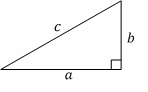##### Right triangles:

A good example of a right triangle is one with a=3, b=4, and c=5, also called a $$3-4-5$$ right triangle. Note that multiples of these numbers are also right triangles. For example, if you multiply these numbers by 2, you get a=6, b=8  and
$$c=10(6-8-10)$$, which is also a right triangle.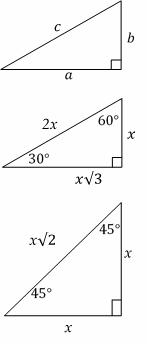##### All triangles: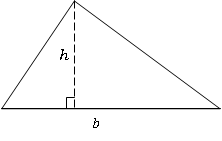Area $$=\frac{1}{2}$$ $$b \times h$$
Angles on the inside of any triangle add up to $$180^\circ$$.
The length of one side of any triangle is always less than the sum and more than the difference of the lengths of the other two sides.
An exterior angle of any triangle is equal to the sum of the two remote interior angles. Other important triangles:

##### Equilateral:

These triangles have three equal sides, and all three angles are $$60^\circ$$.

##### Isosceles:

An isosceles triangle has two equal sides. The “base” angles (the ones opposite the two sides) are equal (see the $$45^\circ$$  triangle above).

### The Most Comprehensive TABE Math Preparation Bundle

$76.99$36.99
52% Off*

Includes TABE Math Prep Books, Workbooks, and Practice Tests

##### Similar:

Two or more triangles are similar if they have the same shape. The corresponding angles are equal, and the corresponding sides are in proportion. For example, the $$3-4-5$$ triangle and the $$6-8-10$$ triangle from before are similar since their sides are in a ratio of $$2$$ to $$1$$.

##### Circles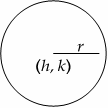Area $$=πr^2$$
Circumference $$=2πr$$
Full circle $$=360^\circ$$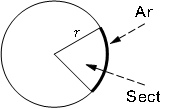Length Of Arc $$= \ \frac{n°}{360°} \times 2πr$$
Area Of Sector $$= \ \frac{n°}{360°} \times πr^2$$

Equation of the circle (above left figure): $$(x \ - \ h)^2 \ + \ (y \ - \ k)^2 \ = \ r^2$$.

##### Rectangles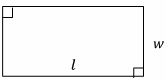(Square if l=w)
Area=lw

##### Parallelogram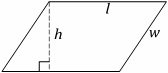(Rhombus if l=w)
Area=lh
Regular polygons are n-sided figures with all sides equal and all angles equal.
The sum of the inside angles of an n-sided regular polygon is
$$(n-2) .180^\circ$$.

##### Area of a parallelogram:

$$A = bh$$

##### Area of a trapezoid:

$$A =\frac{1}{2} \ h (b_{1}+b_{2})$$

##### Surface Area and Volume of a rectangular/right prism:

$$SA=ph+2B$$
$$V=Bh$$

##### Surface Area and Volume of a cylinder:

$$SA =2πrh+2πr^2$$
$$V =πr^2 h$$

##### Surface Area and Volume of a Pyramid

$$SA=\frac{1}{2} \ ps+b$$
$$V=\frac{1}{3}\ bh$$

##### Surface Area and Volume of a Cone

$$SA =πrs+πr^2$$
$$V=\frac{1}{3} \ πr^2 \ h$$

##### Surface Area and Volume of a Sphere

$$SA =4πr^2$$
$$V =\frac{4}{3} \ πr^3$$
(p $$=$$ perimeter of base B; $$π ~ 3.14$$)

##### Solids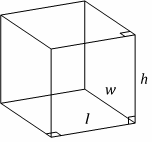Rectangular Solid
Volume $$= \ l \times w \times h$$
Area $$= \ 2(lw \ + \ wh \ + \ lh)$$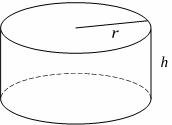Right Cylinder
Volume $$= \ πr^2 \ h$$
Area $$= \ 2πr \ (r \ + \ h)$$

$$x=\frac{-b±\sqrt{b^2-4ac}}{2a}$$

##### Simple interest:

$$I=prt$$
(I = interest, p = principal, r = rate, t = time)

##### mean:

mean: $$\frac{sum \ of \ the \ data}{of \ data \ entires}$$

##### mode:

value in the list that appears most often

##### range:

largest value $$-$$ smallest value

##### Median

Middle value in the list (which must be sorted)
Example: median of
$$\{3,10,9,27,50\} = 10$$
Example: median of
$$\{3,9,10,27\}=\frac{(9+10)}{2}=9.5$$

##### Sum

average $$×$$ (number of terms)

##### Average

$$\frac{sum \ of \ terms}{number \ of \ terms}$$

##### Average speed

$$\frac{total \ distance}{total \ time}$$

##### Probability

$$\frac{number \ of \ desired \ outcomes}{number \ of \ total \ outcomes}$$
The probability of two different events A and B both happening is:
P(A and B)=p(A) .p(B)
as long as the events are independent (not mutually exclusive).

##### Powers, Exponents, Roots

$$x^a .x^b=x^{a+b}$$
$$\frac{x^a}{x^b} = x^{a-b}$$
$$\frac{1}{x^b }= x^{-b}$$
$$(x^a)^b=x^{a.b}$$
$$(xy)^a= x^a .y^a$$
$$x^0=1$$
$$\sqrt{xy}=\sqrt{x} .\sqrt{y}$$
$$(-1)^n=-1$$, if n is odd.
$$(-1)^n=+1$$, if n is even.
If $$0<x<1$$, then
$$0<x^3<x^2<x<\sqrt{x}<\sqrt{3x}<1$$.

##### Simple Interest

The charge for borrowing money or the return for lending it.
Interest = principal $$×$$ rate $$×$$ time
OR
$$I=prt$$

##### Compound Interest

Interest computed on the accumulated unpaid interest as well as on the original principal.
A $$=P(1+r)^t$$
A= amount at end of time
P= principal (starting amount)
r= interest rate (change to a decimal i.e. $$50\%=0.50$$)
t= number of years invested

##### Positive Exponents

An exponent is simply shorthand for multiplying that number of identical factors. So $$4^3$$ is the same as (4)(4)(4), three identical factors of 4. And $$x^3$$ is just three factors of x, $$(x)(x)(x)$$.

##### Negative Exponents

A negative exponent means to divide by that number of factors instead of multiplying.
So $$4^{-3}$$ is the same as $$\frac{1}{4^3}$$ and
$$x^{-3}=\frac{1}{x^3}$$

##### Factorials

Factorial- the product of a number and all counting numbers below it.
8 factorial $$=8!=$$
$$8×7×6×5×4×3×2×1=40,320$$
5 factorial $$=5!=$$
$$5×4×3×2×1=120$$
2 factorial $$=2!=2× 1=2$$

##### Multiplying Two Powers of the SAME Base

When the bases are the same, you find the new power by just adding the exponents
$$x^a .x^b=x^{a+b }$$

##### Multiplying Two Powers of Different Bases Same Exponent

If the bases are different but the exponents are the same, then you can combine them
$$x^a \times y^a \ = \ (xy)^a$$

##### Powers of Powers

For power of a power: you multiply the exponents.
$$(x^a)^b=x^{(ab)}$$

##### Dividing Powers

$$\frac{x^a}{x^b} =x^a x^{-b}= x^{a-b}$$

##### The Zero Exponent

Anything to the 0 power is 1.
$$x^0= 1$$

### TABE Math for Beginners

$24.99$14.99
40% Off*

The Ultimate Step by Step Guide to Preparing for the TABE 11 & 12 Math Level D Test

##### Permutation:

When different orderings of the same items are counted separately, we have a permutation problem:
$$_{n}P_{r}=\frac{n!}{(n-1)!}$$

##### Combination:

The fundamental counting principle, as demonstrated above, is used any time the order of the outcomes is important.  When selecting objects from a group where the order is NOT important, we use the formula for COMBINATIONS:
The fundamental counting principle, as demonstrated above, is used any time the order of the outcomes is important. When selecting objects from a group where the order is NOT important, we use the formula for COMBINATIONS:
$$_{n}C_{r}=\frac{n!}{r!(n-1)!}$$

## More Articles

### The Most Comprehensive FTCE Math Preparation Bundle

$76.99$34.99

### Praxis Core Math for Beginners

$21.99$14.99

$6.99$4.99
$6.99$6.99